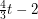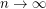Importance: Medium ✭✭
 Author(s): Reed, Bruce A. Wood, David R.
 Subject: Graph Theory » Basic Graph Theory » » Minors
 Keywords: minors
 Posted by: David Wood on: March 16th, 2014
Conjecture   Every graph with average degree at leastcontains every 2-regular graph onvertices as a minor.

Reed and Wood [RW] explained that a result of Corradi and Hajnal [CH] implies that ifis the graph consisting ofdisjoint triangles, then every graph with average degree at leastcontainsas a minor. Moreover, the bound ofis best possible since the complete bipartite graphcontains no-minor, but has average degree tending to(as). Thus the conjecture would generalise this result.

Update: There has been a lot of recent progress on this conjecture [HW,CNLWY].

## Bibliography

[CH] Keresztely Corradi and Andras Hajnal. On the maximal number of independent circuits of a graph. Acta Math. Acad. Sci. Hungar., 14:423–443, 1963.

*[RW] Bruce Reed and David R. Wood. Forcing a sparse minor, arXiv:1402.0272, 2013.

[HW] Daniel J. Harvey and David R. Wood. Cycles of given size in a dense graph. SIAM J. Discrete Math. 29.4:2336–2349, 2015.

[CNLWY] E. Csóka, S. Norin, I. Lo, H. Wu and L. Yepremyan. The extremal function for disconnected minors. J. Comb. Theory B 126 (2017), 162-174.

* indicates original appearance(s) of problem.